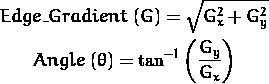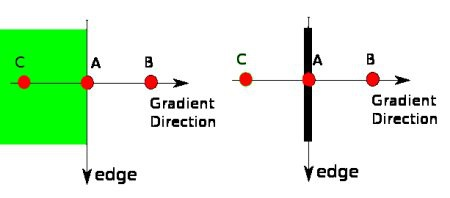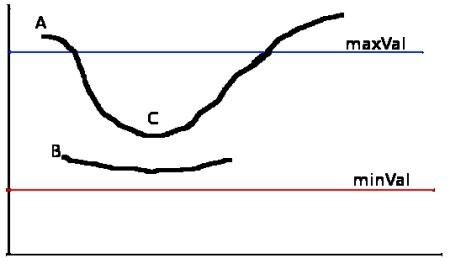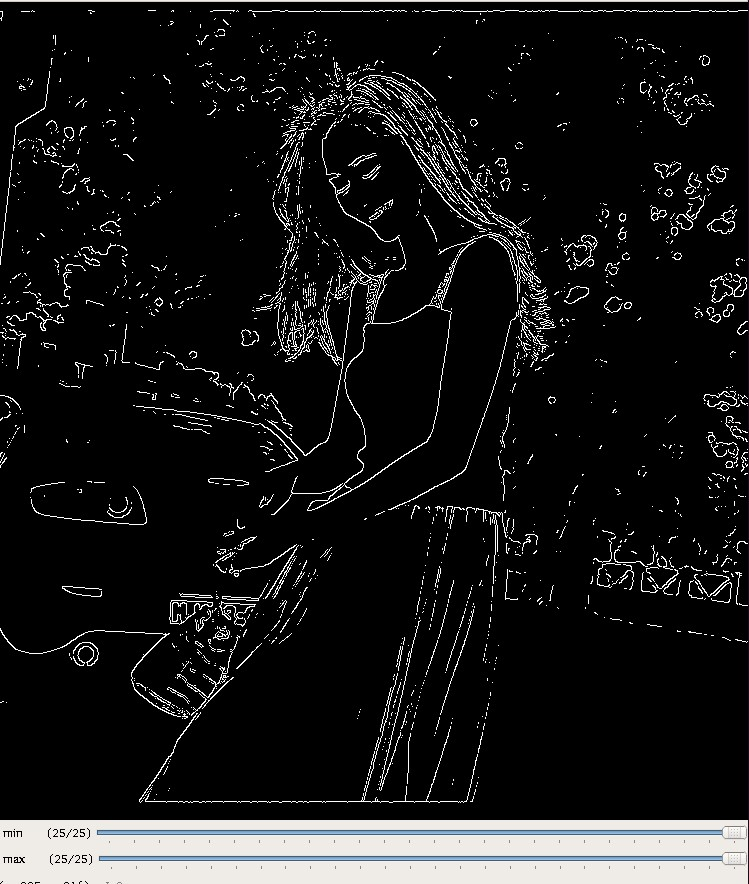Canny Edge Detection

## 1. Canny 边缘检测理论

Canny 是一种常用的边缘检测算法. 其是在 1986 年 John F.Canny 提出的.
Canny 是一种 multi-stage 算法，分别如下：

•  - 应用高斯滤波来平滑图像，去除噪声
•  - 应用非最大抑制(non-maximum suppression)技术来消除边缘误检(本来不是但检测出来是)
•  - 应用双阈值的方法来决定可能的(潜在)边界
•  - 利用滞后技术来跟踪边界

### 1.2 计算图像强度梯度### 1.3 非最大值抑制 NMS### 1.4 滞后阈值(Hysteresis thresholding)## 2. OpenCV 之 Canny 边缘检测

OpenCV 提供了 cv2.canny() 函数.

edge = cv2.Canny(image, threshold1, threshold2[, edges[, apertureSize[, L2gradient ]]])
• 参数 Image - 输入图片，必须为单通道的灰度图
• 参数 threshold1 和 threshold2 - 分别对应于阈值 minVal 和 maxVal
• 参数 apertureSize - 用于计算图片提取的 Sobel kernel 尺寸. 默认为 3.
• 参数 L2gradient - 指定计算梯度的等式. 当参数为 True 时，采用 1.2 中的梯度计算公式，其精度更高；否则采用的梯度计算公式为：${ {Edge\_ Gradient} (G) = |G_x| + |G_y| }$. 该参数默认为 False.

    import cv2
import numpy as np
from matplotlib import pyplot as plt

edges = cv2.Canny(img, 100, 200)

plt.subplot(121),plt.imshow(img,cmap = 'gray')
plt.title('Original Image'), plt.xticks([]), plt.yticks([])
plt.subplot(122),plt.imshow(edges,cmap = 'gray')
plt.title('Edge Image'), plt.xticks([]), plt.yticks([])

plt.show()import numpy as np
import cv2

def nothing(x):
pass

cv2.namedWindow('res')
cv2.createTrackbar('min','res',0,25,nothing)
cv2.createTrackbar('max','res',0,25,nothing)
while(1):
if cv2.waitKey(1)&amp;0xFF==27:
break
maxVal=cv2.getTrackbarPos('max','res')
minVal=cv2.getTrackbarPos('min','res')
canny=cv2.Canny(img,10*minVal,10*maxVal)
cv2.imshow('res',canny)
cv2.destroyAllWindows()opencv-python(13):Canny边缘检测

## 3. OpenCV-Python-Tutorial 之 Canny边缘检测

Canny.py

# -*- coding: utf-8 -*-

'''
Canny 边缘检测是一种非常流行的边缘检测算法，是由John F.Canny 在
1986 年提出的. 它是一个有很多步构成的算法.

OpenCV 中的 Canny 边界检测

'''

import cv2
import numpy as np
from matplotlib import pyplot as plt

edges = cv2.Canny(img, 100, 200)

cv2.imshow('Edges',edges)
cv2.waitKey(0)

# plt.subplot(121), plt.imshow(img, cmap='gray')
# plt.title('Original Image'), plt.xticks([]), plt.yticks([])
# plt.subplot(122), plt.imshow(edges, cmap='gray')
# plt.title('Edge Image'), plt.xticks([]), plt.yticks([])
# plt.show()


## Related

Last modification：October 12th, 2018 at 09:20 am